flummoxed (again!)

allegansveritatem

Full Member
Here is the problem: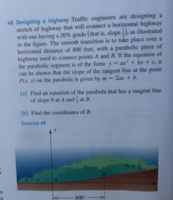I don't recall ever being introduced to this business of tangent lines whose slopes can be used to find coordinates of points on a parabola. I spent about one and a half hours digging around and trying this and that but could not quite get a handle on the matter. I was able to figure out, by the fact that the one tangent line has a slope of 0 that the vertex of this parabola is (0,0), but could not see how to use the 1/5 slope to any advantage. I tried using it in a slope formula but had two unknowns and couldn't get any further. Here is some of what I tried: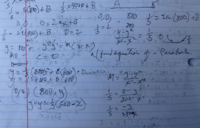So, as the feller said."What to do?"

Dr.Peterson

Elite Member
You seem to have skipped over finding b, which should have come very early. You also did a lot with the equation of the line, which you don't have to do. You've also used B instead of b, which are very different things here. In general, it's hard to guess exactly what you were thinking.

I would start by just writing equations for the given conditions:

Passes through (0,0) with slope 0.​
Passes through (800,?) with slope 1/5.​

These give four equations! The first will determine c; the second, b. The fourth will determine c, and the third will answer part (b).

Write out the equations first, and show us what you have. Only then should you start solving.

JeffM

Elite Member
Personally, I find this a deceptive question. Basic calculus says

$$\displaystyle \text {the slope of the parabola of } ax^2 + bx + c \text { at x} = 2ax + b.$$

$$\displaystyle f(x) = ax^2 + bx + c \implies f'(x) = 2ax + b \text { for all x.}$$

Thus, this no clue at all. It is just, for those who have not learned differential calculus, a given.

Moreover, they have (quite sensibly) picked x = 0 to be the point where the slope = 0, and x = 800 to be the point where the slope = 0.2. So this is a very simple algebra problem

allegansveritatem

Full Member
You seem to have skipped over finding b, which should have come very early. You also did a lot with the equation of the line, which you don't have to do. You've also used B instead of b, which are very different things here. In general, it's hard to guess exactly what you were thinking.

I would start by just writing equations for the given conditions:

Passes through (0,0) with slope 0.​
Passes through (800,?) with slope 1/5.​

These give four equations! The first will determine c; the second, b. The fourth will determine c, and the third will answer part (b).

Write out the equations first, and show us what you have. Only then should you start solving.
OK. I will give it a go tomorrow and see what I can do. Thanks

allegansveritatem

Full Member
Personally, I find this a deceptive question. Basic calculus says

$$\displaystyle \text {the slope of the parabola of } ax^2 + bx + c \text { at x} = 2ax + b.$$

$$\displaystyle f(x) = ax^2 + bx + c \implies f'(x) = 2ax + b \text { for all x.}$$

Thus, this no clue at all. It is just, for those who have not learned differential calculus, a given.

Moreover, they have (quite sensibly) picked x = 0 to be the point where the slope = 0, and x = 800 to be the point where the slope = 0.2. So this is a very simple algebra problem
I don't say it is intrinsically a difficult problem, but in the context of my relative ignorance, well...it ain't so easy.

JeffM

Elite Member
I did not mean to imply that you should find the question easy. I meant that the problem is designed to lead you astray, and I do not like problems like that. In terms of what you are being asked to do, the problem is one of simultaneous linear equations. As for the coordinates of B, there is nothing to solve in the x dimension. Nor are x and y ever explicitly defined. Ignoring the non-mathematical material, here is the problem reworded less deceptively.

x is the horizontal distance in feet from point A. y is the vertical distance in feet above point A. The horizontal distance between A and B is 800 feet. Joining A and B is a parabola such that

$$\displaystyle y = ax^2 + bx + c \text { with a slope of } 0 \text { and } 0.2 \text { at A and B respectively.}$$

The equation describing the slope of that parabola is $$\displaystyle 2ax + b.$$

Find the coordinates of B.

Now for some hints.

Without any algebra at all, what is the numeric value of the x coordinate of point B?

What is the algebraic expression for the y coordinate of point B?

What then are the unknowns that you must find?

To find that many unknowns, you need how many equations?

What are they?

JeffM

Elite Member
I do not mind problems that require work and creativity to turn them into a mathematically tractable form. I do mind problems where basic information, such as the definitions of x and y, are never explicitly given.

allegansveritatem

Full Member
I did not mean to imply that you should find the question easy. I meant that the problem is designed to lead you astray, and I do not like problems like that. In terms of what you are being asked to do, the problem is one of simultaneous linear equations. As for the coordinates of B, there is nothing to solve in the x dimension. Nor are x and y ever explicitly defined. Ignoring the non-mathematical material, here is the problem reworded less deceptively.

x is the horizontal distance in feet from point A. y is the vertical distance in feet above point A. The horizontal distance between A and B is 800 feet. Joining A and B is a parabola such that

$$\displaystyle y = ax^2 + bx + c \text { with a slope of } 0 \text { and } 0.2 \text { at A and B respectively.}$$

The equation describing the slope of that parabola is $$\displaystyle 2ax + b.$$

Find the coordinates of B.

Now for some hints.

Without any algebra at all, what is the numeric value of the x coordinate of point B?

What is the algebraic expression for the y coordinate of point B?

What then are the unknowns that you must find?

To find that many unknowns, you need how many equations?

What are they?
I appreciate this info. I worked the thing out today before reading this but I will read it over tomorrow when l am in math mode and I will absorb what I can.

allegansveritatem

Full Member
OK. I will give it a go tomorrow and see what I can do. Thanks
I went at it again today and I worked it out to a satisfactory conclusion although not always by strictly algebraic means. I will post my procedure but would like to say a few things about it first. I took Dr P's hint and went looking for equations. I didn't find 4 however. And one of the equations, the equation of the line with slope 1/5 was found by guessing from the diagram what the valve was of the x intercept of said line. In this case I happened to guess right. Later (an Archimedes moment) while using the slope formula to test my results I realized that I didn't need to guess from the diagram, I could have set up the formula and plugged x values in from , say, 300 on and soon would have come to the right one. So here is how I did it and any comments on my methods would be appreciated.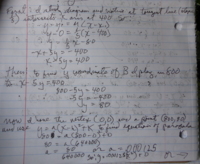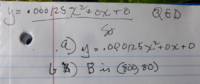I forgot to include in the conclusion the equation of tangent line which is: x-5y=400

Dr.Peterson

Elite Member
Never base your work on something you guess from what a picture looks like! Yes, it does turn out that the line's x-intercept is at 400, because of a special property of the parabola; and your answer is correct; but by even looking at a guessed fact, you were wasting your time (and missing the point of the exercise)!

Here is my hint (with a correction):
I would start by just writing equations for the given conditions:

Passes through (0,0) with slope 0.​
Passes through (800,?) with slope 1/5.​

These give four equations! The first will determine c; the second, b. The fourth will determine a, and the third will answer part (b).
You are told to work with the parabola in the form y = ax^2 + bx + c, and you are told (because, I presume, you haven't learned calculus) that the slope at a given x is 2ax + b.

First equation: Passes through (0,0): a(0)^2 + b(0) + c = 0​
Second equation: Slope at x=0 is 0: 2a(0) + b = 0​
Third equation: Passes through (800,?): a(800)^2 + b(800) + c = answer to part (b)​
Fourth equation: Slope at x=800 is 1/5: 2a(800) + b = 0.2​

Now simplify, then solve the first equation for c, the second for b, and then the fourth for a. That will give you the equation.

allegansveritatem

Full Member
Yes, I know that the diagram guess was a fluke. But I was feeling stuck at that point. Later, as I said, I found another way to use the slope formula to find the x intercept...there was still an element of guessing involved but it was of a more respectable sort. But I will study over your equations and work them out and post the results.

JeffM

Elite Member
If you go back to my second post, you will see I asked a set of questions.

The x coordinate of B is 800. That info is indirectly given in the problem itself. (Assuming you set up your coordinate system with A as the origin, an assumption not explicitly stated in the problem. That is the creativity that this problem can elicit. When I said I did not like the problem, what I was referring to was its presentation rather than the ideas behind it.)

The y coordinate of B is therefore expressed algebraically as:

$$\displaystyle a *800^2 + 800b + c.$$

To evaluate that requires finding 3 unknowns, namely a, b, and c.

So you need 3 equations. With some thought, you should figure out that you know the coordinates of point A and the slope at A plus the slope at B plus you are given the equation of the slope at any point from A to B. These give you three equations.

$$\displaystyle a * 0^2 + b * 0 + c = 0,\ 2a * 0 + b = 0, \text { and } 2a * 800 + b = 0.2.$$

From the first equation, it is trivial to find that c = 0. From the second equation it is trivial to find that b = 0. So the third equation reduces to

$$\displaystyle 2a * 800 = 0.2 \implies 1600a = 0.2 = 2000 * 10^{-4} \implies 4a = 5 * 10^{-4} \implies a = \dfrac{5}{4} * 10^{-4}.$$

Now going back to our expression for the y coordinate of point B

$$\displaystyle a * 800^2 + 0 * 800 + 0 = \dfrac{5}{4} * 10^{-4} * (8^2 * 10^2)^2 = \dfrac{5}{4} * 64 * 10^{-4} * 10^4 = 5 * 16 = 80.$$

There is no need to guess anything.

It was a useful exercise in setting up a coordinate system and finding the coeffecients of a non-linear function using simultaneous linear equations, but the presentation left a lot to be desired.

EDIT: In real world applications of mathematics, you do sometimes have to guess because not all the information required mathematically is available. That creates extra-mathematical issues, especially in terms of presentation of conclusions. But in terms of mathematics itself, no guessing is allowed.

Last edited:

Dr.Peterson

Elite Member
It's worth noting that the picture, which is explicitly referred to in the problem, defines the coordinate system. It would be better if this were explicitly stated in words (to get students used to how such things are said, and also used to not assuming anything from a picture), but that does make the problem reasonable, in my mind.

•JeffM

JeffM

Elite Member
It's worth noting that the picture, which is explicitly referred to in the problem, defines the coordinate system. It would be better if this were explicitly stated in words (to get students used to how such things are said, and also used to not assuming anything from a picture), but that does make the problem reasonable, in my mind.
I suppose the issue lies in the level of spophistication expected of the students for which the text is intended. It seemed to me that the OP was basically in the dark because so much was not stated in words.

allegansveritatem

Full Member
If you go back to my second post, you will see I asked a set of questions.

The x coordinate of B is 800. That info is indirectly given in the problem itself. (Assuming you set up your coordinate system with A as the origin, an assumption not explicitly stated in the problem. That is the creativity that this problem can elicit. When I said I did not like the problem, what I was referring to was its presentation rather than the ideas behind it.)

The y coordinate of B is therefore expressed algebraically as:

$$\displaystyle a *800^2 + 800b + c.$$

To evaluate that requires finding 3 unknowns, namely a, b, and c.

So you need 3 equations. With some thought, you should figure out that you know the coordinates of point A and the slope at A plus the slope at B plus you are given the equation of the slope at any point from A to B. These give you three equations.

$$\displaystyle a * 0^2 + b * 0 + c = 0,\ 2a * 0 + b = 0, \text { and } 2a * 800 + b = 0.2.$$

From the first equation, it is trivial to find that c = 0. From the second equation it is trivial to find that b = 0. So the third equation reduces to

$$\displaystyle 2a * 800 = 0.2 \implies 1600a = 0.2 = 2000 * 10^{-4} \implies 4a = 5 * 10^{-4} \implies a = \dfrac{5}{4} * 10^{-4}.$$

Now going back to our expression for the y coordinate of point B

$$\displaystyle a * 800^2 + 0 * 800 + 0 = \dfrac{5}{4} * 10^{-4} * (8^2 * 10^2)^2 = \dfrac{5}{4} * 64 * 10^{-4} * 10^4 = 5 * 16 = 80.$$

There is no need to guess anything.

It was a useful exercise in setting up a coordinate system and finding the coeffecients of a non-linear function using simultaneous linear equations, but the presentation left a lot to be desired.

EDIT: In real world applications of mathematics, you do sometimes have to guess because not all the information required mathematically is available. That creates extra-mathematical issues, especially in terms of presentation of conclusions. But in terms of mathematics itself, no guessing is allowed.
Yes, I see this now. What you are saying is basically what Dr P says. I worked through his suggested equations and will put up my results. As for guessing...I will defend my procedure (when I was guessing) to this extent: I tested the results to make sure my guess was correct. My method in this case was a little like adjusting the trajectory of a cannon--you fire it and have somebody in a balloon tell you how far off you are, You keep adjusting til you get it right. That worked fine in this problem mainly because there was a very limited range of values to choose from. If the range had been huge, I would have been searching hay stacks for needles. But, as you say, why guess when there is a more efficient way? Or, why study algebra if you would rather waste your time guessing?

allegansveritatem

Full Member
Never base your work on something you guess from what a picture looks like! Yes, it does turn out that the line's x-intercept is at 400, because of a special property of the parabola; and your answer is correct; but by even looking at a guessed fact, you were wasting your time (and missing the point of the exercise)!

Here is my hint (with a correction):

You are told to work with the parabola in the form y = ax^2 + bx + c, and you are told (because, I presume, you haven't learned calculus) that the slope at a given x is 2ax + b.

First equation: Passes through (0,0): a(0)^2 + b(0) + c = 0​
Second equation: Slope at x=0 is 0: 2a(0) + b = 0​
Third equation: Passes through (800,?): a(800)^2 + b(800) + c = answer to part (b)​
Fourth equation: Slope at x=800 is 1/5: 2a(800) + b = 0.2​

Now simplify, then solve the first equation for c, the second for b, and then the fourth for a. That will give you the equation.
I worked through these problems today. I think I have a pretty clear idea now of what was being asked for in this problem. I can say in defence of my initial bewilderment that this method was not explicitly outlined in the text. What we were shown is how to find the equation of a parabola if the vertex and one other point on the parabola is known. but I see now that this problem is just a special case of that situation.
One thing: the third equation did not result in a definitive result for me because I still didn't know what a was until I worked the last problem. Then I could go back and finish the third equation.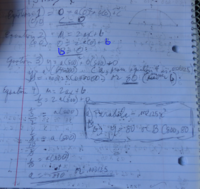Dr.Peterson

Elite Member
Looks good, though it's a little hard to read.

I often wish I could look over a student's shoulder as I do in person, and see what they have been learning. If you had mentioned learning to find a quadratic given the vertex and another point, I might have asked you to show how you do that, and then pointed out that the same method can be adapted to this problem, as you indicate.

You should not expect to be told a specific method for solving a problem; in the adult world, that's how you get low pay! Real work means figuring out for yourself how to do something you haven't seen before, using the tools you have (or inventing a new one). That's why a good teacher stretches you with new problems after you've mastered routine problems.

JeffM

Elite Member
I have quite a few observations to make.

First, yes my method and Dr. P's methods are virtually identical in terms of the algebra involved. The initial thought process, however, was a bit different. He started by writing equations for the known facts. That is always a GREAT idea. I started by defining my variables and coordinate system, which is another good idea. (Actually Dr. P had to define his variables and coordinate sysytem before he could enter numerical values into his four equations, but he did his defining implicitly, probably automatically.) There is no theoretical difference between implicit and explicit definition, but I have found that writing down the definition of my variables as the very first thing I always do frequently helps me organize my thoughts when trying to tackle a problem. In this case, such a definition leads very directly to the choice of a coordinate system with point A being the origin. And that choice immediately gives you the x-coordinate of B without any further work. This difference in initial work is why Dr. P came up with four equations, but I needed only three.

Second, the problem is soluble no matter what coordinate system you choose, but the computations are MUCH easier by choosing point A as the origin. Dr. P and I both probably "saw" that without any conscious thought. That is a matter of experience, but obviously computations with zero are going to be easy arithmetically.

Third, Dr. P and I are quite familiar with a class of problems that involves finding the coefficients of a known or suspected type of non-linear function by solving a system of linear equations. You are not so there is no wonder that you found this problem difficult. What I still find mysterious is why you had difficulty with the simultaneous linear equations: you should be quite familiar with that class of problem.

Lastly, I realized that I said something misleading before. The method of successive approximations is a perfectly valid mathematical method of finding approximate answers. And sometimes finding an approximate answer is the best that you can do. But exact answers are highly preferred over approximations, and when you give an approximation it is usually important to put a bound on the potential error. So I was too dogmatic about guessing. For example, Newton's method of finding approximate roots starts with an initial guess. The topic of such methods is covered in courses on numerical methods.

Last edited:

allegansveritatem

Full Member
Looks good, though it's a little hard to read.

I often wish I could look over a student's shoulder as I do in person, and see what they have been learning. If you had mentioned learning to find a quadratic given the vertex and another point, I might have asked you to show how you do that, and then pointed out that the same method can be adapted to this problem, as you indicate.

You should not expect to be told a specific method for solving a problem; in the adult world, that's how you get low pay! Real work means figuring out for yourself how to do something you haven't seen before, using the tools you have (or inventing a new one). That's why a good teacher stretches you with new problems after you've mastered routine problems.
Yes, right. The real world is like a coloring book with half the lines erased--half, that is, if you're lucky.

allegansveritatem

Full Member
I have quite a few observations to make.

First, yes my method and Dr. P's methods are virtually identical in terms of the algebra involved. The initial thought process, however, was a bit different. He started by writing equations for the known facts. That is always a GREAT idea. I started by defining my variables and coordinate system, which is another good idea. (Actually Dr. P had to define his variables and coordinate sysytem before he could enter numerical values into his four equations, but he did his defining implicitly, probably automatically.) There is no theoretical difference between implicit and explicit definition, but I have found that writing down the definition of my variables as the very first thing I always do frequently helps me organize my thoughts when trying to tackle a problem. In this case, such a definition leads very directly to the choice of a coordinate system with point A being the origin. And that choice immediately gives you the x-coordinate of B without any further work. This difference in initial work is why Dr. P came up with four equations, but I needed only three.

Second, the problem is soluble no matter what coordinate system you choose, but the computations are MUCH easier by choosing point A as the origin. Dr. P and I both probably "saw" that without any conscious thought. That is a matter of experience, but obviously computations with zero are going to be easy arithmetically.

Third, Dr. P and I are quite familiar with a class of problems that involves finding the coefficients of a known or suspected type of non-linear function by solving a system of linear equations. You are not so there is no wonder that you found this problem difficult. What I still find mysterious is why you had difficulty with the simultaneous linear equations: you should be quite familiar with that class of problem.

Lastly, I realized that I said something misleading before. The method of successive approximations is a perfectly valid mathematical method of finding approximate answers. And sometimes finding an approximate answer is the best that you can do. But exact answers are highly preferred over approximations, and when you give an approximation it is usually important to put a bound on the potential error. So I was too dogmatic about guessing. For example, Newton's method of finding approximate roots starts with an initial guess. The topic of such methods is covered in courses on numerical methods.
When you say"simultaneous linear equations" do you mean what is called "systems of equations"? If so, yes, I am familiar with that procedure but did not recognize it here....but I guess that is exactly what is going on here, no?